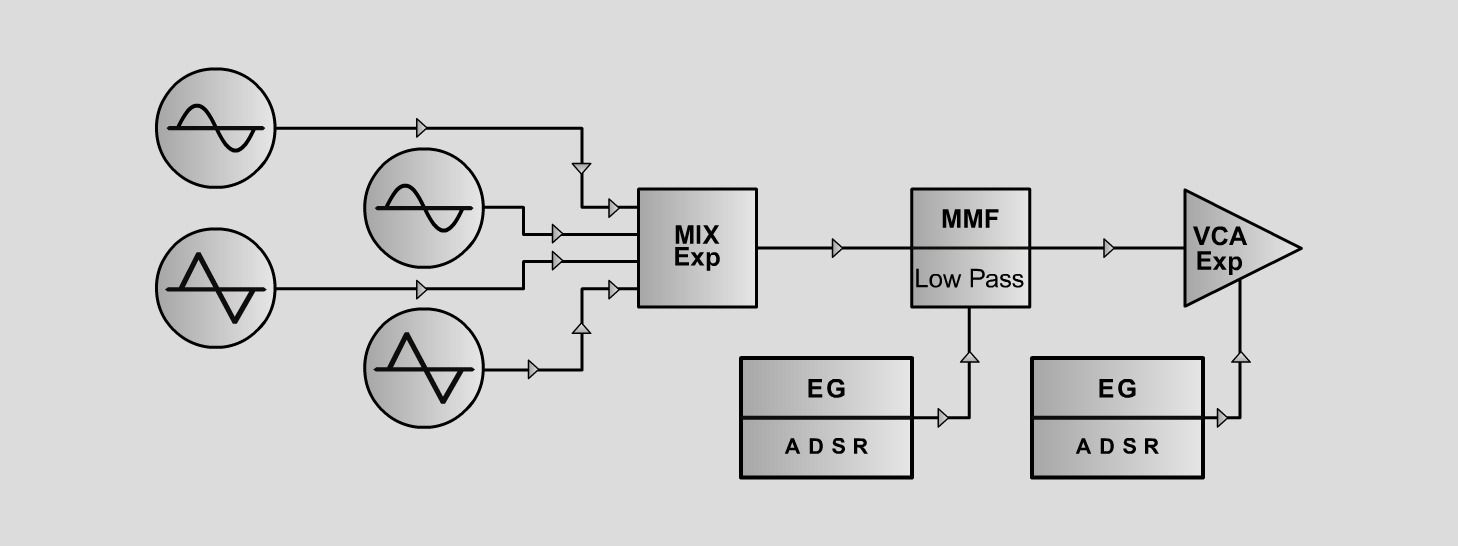Solar-FluteInstrumentsD o e p f e r A - 100 Connections: Settings: Solar-Flute 1: A-111/1 (Sine)  <=>  A-138exp (Input 1)   A-111/2 (Sine)  <=>  A-138exp (Input 2)   A-111/3 (Triangle)  <=>  A-138exp (Input 3)   A-111/4 (Triangle)  <=>  A-138exp (Input 4)   A-138exp (Output) <=>  A-121 (Audio In)   A-121 (Low) <=>  A-131 (Audio In 1)   A-140/1 (Output)  <=>   A-121 (FCV2)   A-140/2 (Output) <=>   A-131 (CV1) A-138exp (In1 = 0, In2 = 5, In3 = 0, In4 = 8, Out = 8)   A-121 (Audio = 8, Frequ = 5.5, FCV2 = 8, Res = 5)   A-140/1 (A = 0.5, D = 3, S = 0, R = 4,   Range = M)   A-140/2 (A = 2, D = 3, S = 10, R = 4,  Range = M)   A-131 (Gain = 0, Audio In 1 = 10, Audio Out = 8) Solar-Flute 2: A-138exp (In1 = 4, In2 = 7, In3 = 2, In4 = 1, Out = 8)   A-121 (Audio = 8, Frequ = 5.5, FCV2 = 8, Res = 5)   A-140/1 (A = 0.5, D = 3, S = 0, R = 4,   Range = M)   A-140/2 (A = 2, D = 3, S = 10, R = 4,  Range = M)   A-131 (Gain = 0, Audio In 1 = 10, Audio Out = 8) Solar-Flute 3: A-138exp (In1 = 3, In2 = 7, In3 = 4, In4 = 10, Out = 7)   A-121 (Audio = 8, Frequ = 5.5, FCV2 = 8, Res = 5)   A-140/1 (A = 0.5, D = 3, S = 0, R = 4,   Range = M)   A-140/2 (A = 2, D = 3, S = 10, R = 4,  Range = M)   A-131 (Gain = 0, Audio In 1 = 10, Audio Out = 8) Notes: Tune A-111/2 and A-111/4 one octave higher Josef MuellerSound samples Bach: Badinerie (BWV 1067) Solar-Flute 1 A Solar-Flute 1 B Solar-Flute 1 C Solar-Flute 2 A Solar-Flute 2 B Solar-Flute 2 C Solar-Flute 3 A Solar-Flute 3 B Solar-Flute 3 C